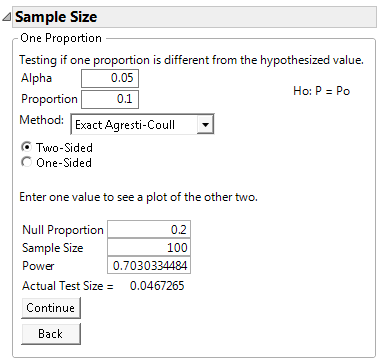Publication date: 04/12/2021

## Example of the One Sample Proportion Calculator

Suppose that an assembly line has a historical proportion of defects equal to 0.1. Given a sample size of 100 and an alpha level of 0.05, you want to calculate the power to detect a defect rate that differs by 0.1 or more from the historical rate.

1. Select DOE > Design Diagnostics > Sample Size and Power.

2. Click One Sample Proportion.

3. Leave Alpha as 0.05.

4. Leave 0.1 as the value for Proportion.

5. Leave the Method as Exact Agresti-Coull.

6. Leave the test type as Two-Sided.

7. Leave 0.2 as the value for Null Proportion.

8. Enter 100 as the Sample Size.

9. Click Continue.

Figure 17.12 One Sample Proportion CalculatorFor a sample size of 100, the power is approximately 70%. The Actual Test Size is approximately 0.0467, which is slightly less than the desired 0.05. With a sample size of 100, if the defect rate is 0.2, the probability of a Type I error of rejecting the null hypothesis is 5%. Alternatively, the probability of a Type II error of not rejecting the null hypothesis is 30% (1 - power).

Want more information? Have questions? Get answers in the JMP User Community (community.jmp.com).